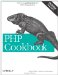# Recipe 4.3. Initializing an Array to a Range of Integers

#### 4.3.1. Problem

You want to assign a series of consecutive integers to an array.

#### 4.3.2. Solution

Use range(\$start, \$stop):

`\$cards = range(1, 52);`

#### 4.3.3. Discussion

For increments other than 1, you can use:

`function pc_array_range(\$start, \$stop, \$step) {     \$array = array();     for (\$i = \$start; \$i <= \$stop; \$i += \$step) {         \$array[] = \$i;     }     return \$array; }`

So for odd numbers:

`\$odd = pc_array_range(1, 52, 2);`

And for even numbers:

`\$even = pc_array_range(2, 52, 2);`

In PHP 5.0.0 and above, you don't need pc_array_range( ): just pass an increment to range( ) as a third argument:

`\$odd = range(1, 52, 2); \$even = range(2, 52, 2);`

Recipe 2.4 for how to operate on a series of integers; documentation on range( ) at http://www.php.net/range.PHP Cookbook: Solutions and Examples for PHP Programmers
ISBN: 0596101015
EAN: 2147483647
Year: 2006
Pages: 445

Similar book on Amazon